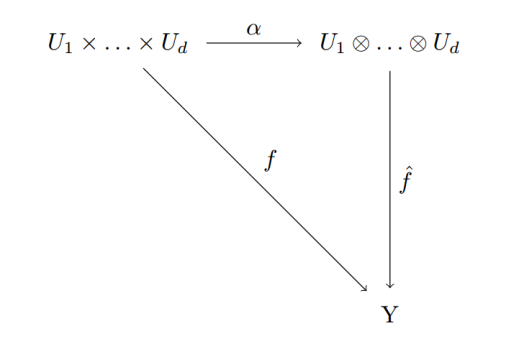Category Theory

# Universal Property of Quotient Vector SpaceDescribe the universal property of quotient vector spaces.

Solution:
Let $$V \in Obj(Vect_{K})$$. Any subspace $$U\subseteq V$$ and its projection $$\pi: V\longrightarrow V/U$$ form a universal pair.

Rephrasing: let any vector space $$W$$ and a linear transformation $$f\in Hom_{Vect_{k}}(V,W)$$ with $$U\subseteq ker(f)$$. There is a unique factoring funtion $$\varphi$$ such that $$\varphi\circ \pi = f$$.

i) Existence. Letting $$\varphi(v+U)=f(v)$$. The function φ is well-defined for any $$v\in V$$. If $$v+U=v’+U$$, we know $$v-v’\in U$$, therfore  $$f(v-v’) = 0$$. Since $$f$$ is linear, $$\varphi(v+U)=f(v)=f(v’)=\varphi(v’+U)$$.

Linearity of $$f$$ gives the same to φ: $$\varphi((v+U)+\alpha(w+U)) = \varphi((v+\alpha w)+U)=f(v+\alpha w)) = f(v)+\alpha f(w)$$.

ii) Uniquiness. The function $$\pi: v\mapsto v+ U$$ is surjective, and any surjective function in the category of sets is an epimorphism, the left cancellation rule holds:

$\varphi\circ\pi = \varphi’\circ\pi \Longrightarrow \varphi = \varphi’.$

Tags: